# Circles Notes Class 11th Maths

0
1683

Circle is defined as the locus of a point which moves in a plane such that its distance from a fixed point in that plane is constant.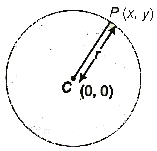Standard Forms of a Circle

(i) Equation of circle having centre (h, k) and radius (x — h)2 + (y — k)2 = a2.

If centre is (0, 0), then equation of circle is x2 + y2 = a2.

(ii) When the circle passes through the origin, then equation of the circle is x2 + y2 — 2hx — 2ky = 0.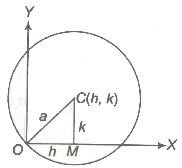(iii) When the circle touches the X-axis, the equation is x2 + y2 — 2hx — 2ay + h2 = O.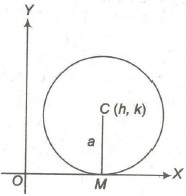(iv) Equation of the circle, touching the Y-axis is x2 + y2 — 2ax — 2ky + k2 = 0.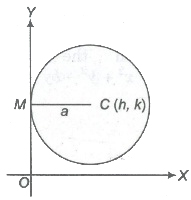(v) Equation of the circle, touching both axes is x2 + y2 — 2ax — 2ay + a2 = O.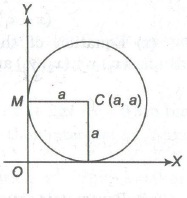(vi) Equation of the circle passing through the origin and centre lying on the X-axis is x2 + y2 — 2ax = O.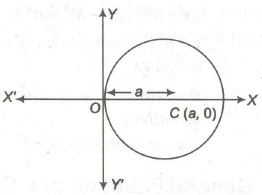(vii) Equation of the circle passing through the origin and centre lying on the Y-axis is x2 + y2 – 2ay = 0.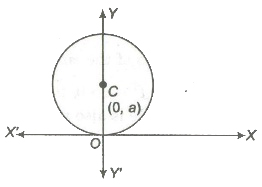(viii) Equation of the circle through the origin and cutting intercepts a and b on the coordinate axes is x2 + y2 — by = 0.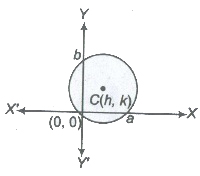(ix) Equation of the circle, when the coordinates of end points of a diameter are (x1, y1) and (x2, y2) is

(x — x1)(x — x2) + (y – y1)(y — y2) = 0.

(x) Equation of the circle passes through three given points (x1, y1), (x2, y2) and (x3, y3) is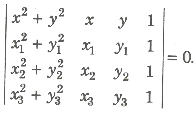(xi) Parametric equation of a circle

(x – h)2 + (y – k)2 = a2 is
x = h + a cosθ, y = k + a sinθ,
0 ≤ θ ≤ 2π
For circle x2 + y2 = a2, parametric equation is
x = a cos θ, y = a sin θ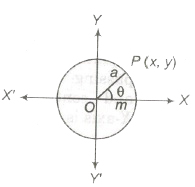General Equation of a Circle

The general equation of a circle is given by x2 + y2 + 2gx + 2fy + c = 0, where centre of the circle = (- g, – f)

Radius of the circle = √g2 + f2 – c

1. If g2 + f2 – c > 0, then the radius of the circle is real and hence the circle is also real.
2. If g2 + f2 – c = 0, then the radius of the circle is 0 and the circle is known as point circle.
3. If g2 + f2 – c< 0, then the radius of the circle is imaginary. Such a circle is imaginary, which is not possible to draw.

Position of a Point with Respect to a Circle

A point (x1, y1) lies outside on or inside a circle

S ≡ x2 + y2 + 2gx + 2fy + c = 0, according as S1 > , = or < 0
where, S1 = x12 + y12 + 2gx1, + 2fy1+ c

Intercepts on the Axes

The length of the intercepts made by the circle x2 + y2 + 2gx + 2fy + c = 0 with X and Y-axes are

2√g2 – c and 2√g2 – c.

1. If g2 > c, then the roots of the equation x2 + 2gx + c = 0 are real and distinct, so the circle x2 + y2 + 2gx + 2fy + c = 0 meets the X-axis in two real and distinct points.
2. If g2 = c, then the roots of the equation x2 + 2gx + c = 0 are real and equal, so the circle touches X-axis, then intercept on X-axis is O.
3. If g2 < c, then the roots of the equation x2 + 2gx + c = 0 are imaginary, so the given circle does not meet X-axis in real point. Similarly, the circle x2 + y2 + 2gx + 2fy + c = 0 cuts the Y-axis in real and distinct points touches or does not meet in real point according to f2 >, = or < c

Equation of Tangent

A line which touch only one point of a circle.

1. Point Form

1. The equation of the tangent at the point P(x1, y1) to a circle x2 + y2 2gx + 2fy + c= 0 is xx1 + yy1 + g(x + x1) + f(y + y1) + c = 0
2. The equation of the tangent at the point P(x1, y1) to a circle x2 + y2 is xx1 + yy1 = r2

2. Slope Form

(i) The equation of the tangent of slope m to the circle x2 + y2 + 2gx + 2fy + c = 0

are y + f = m(x + g) ± √(g2 + f2 — c)(1 + m2)

(ii) The equation of the tangents of slope m to the circle (x – a)2 (y – b)2 = r2 are y – b = m(x – a) ± r√(1 + m2) and the coordinates of the points of contact are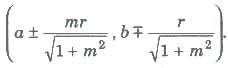(iii) The equation of tangents of slope m to the circle x2 + y2 = r2 are y = mx ± r√(1 + m2)and the coordinates of the point of contact are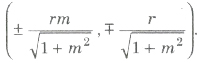3. Parametric Form

The equation of the tangent to the circle (x – a)2 + (y – b)2 = r2 at the point (a + r cos θ, b + r sinθ) is (x – a) cos θ + (y – b) sin θ = r.

Equation of Normal

A line which is perpendicular to the tangent.

1. Point Form

1. (i) The equation of normal at the point (x1, y1) to the circle x2 + y2 + 2gx + 2fy + c = 0 is
y – y1 = [(y1 + f)(x – x1)]/(x1 + g)
(y1 + f)x – (x1 + g)y + (gy1 – fx1) = 0
2. (ii) The equation of normal at the point (x1, y1) to the circle
x2 + y2 = r2 is x/x1 = y/y1

2. Parametric Form

The equation of normal to the circle x2 + y2 = r2 at the point (r cos θ, r sin θ) is

(x/r cos θ) = (y/r sin θ)

or y = x tan θ.

Important Points to be Remembered

(i) The line y = mx + c meets the circle in unique real point or touch the circle

x2 + y2 + r2, if r = |c/√1 + m2

and the point of contacts are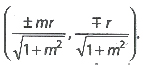(ii) The line lx + my + n = 0 touches the circle x2 + y2 = r2, if r2(l2 + m2) = n2.

(iii) Tangent at the point P (θ) to the circle x2 + y2 = r2 is x cos θ + y sin θ = r.

(iv) The point of intersection of the tangent at the points P(θ1) and Q(θ2) on the circle x2 + y2 = r2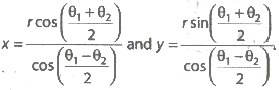(v) Normal at any point on the circle is a straight line which is perpendicular to the tangent to the curve at the point and it passes through the centre of circle.

(vi) Power of a point (x1, y1) with respect to the circle x2 + y2 + 2gx + 2fy + c = 0 is x12 + y12 + 2gx1 + 2fy1 + c.

(vii) If P is a point and C is the centre of a circle of radius r, then the maximum and minimum distances of P from the circle are CP + r and CP — r , respectively.

(viii) If a line is perpendicular to the radius of a circle at its end points on the circle, then the line is a tangent to the circle and vice-versa.

Pair of Tangents

(i) The combined equation of the pair of tangents drawn from a point P(x1, y1) to the circle x2 + y2 = r2 is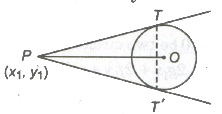(x2 + y2 – r2)(x12+ y12 – r12) = (xx1 + yy1 – r2)2
or SS1 = T2
where, S = x2 + y2 – r2, S1 = x12+ y12 – r12
and T = xx1 + yy1 – r2

(ii) The length of the tangents from the point P(x1, y1) to the circle x2 + y2 + 2gx + 2fy + c = 0 is equal to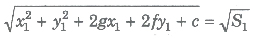(iii) Chord of contact TT’ of two tangents, drawn from P(x1, y1) to the circle x2 + y2 = r2 or T = 0.

Similarly, for the circle

x2 + y2 + 2gx + 2fy + c = 0 is

xx1 + yy1 + g(x + x1) + f(y + y1) + c = 0

(iv) Equation of Chord Bisected at a Given Point The equation of chord of the circle S ≡ x2 + y2 + 2gx + 2fy + c = 0 bisected at the point (x1, y1) is give by T = S1.
i.e., xx1 + yy1 + g (x + x1) + f (y + y1) + c
= x12 + y12 + 2gx1 + fy1 + c

(v) Director Circle The locus of the point of intersection of two perpendicular tangents to a given circle is called a director circle. For circle x2 + y2 = r2, the equation of director circle is x2 + y2 = 2r2.

Common Chord

The chord joining the points of intersection of two given circles is called common chord.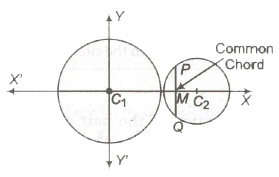(i) If S1 = 0 and S1 = 0 be two circles, such that

S1 ≡ x2 + y2 + 2g1x + 2f1y + c1 = 0
and S2 ≡ x2 + y2 + 2g2x + 2f2y + c2 = 0
then their common chord is given by S1 — S2 = 0

(ii) If C1, C2 denote the centre of the given circles, then their common chord

PQ = 2 PM = 2√(C1P)2 – C1M)2

(iii) If r1, and r2 be the radii of ‘two circles, then length of common chord is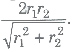Angle of Intersection of Two Circles

The angle of intersection of two circles is defined as the angle between the tangents to the two circles at their point of intersection is given by

cos θ = (r12 + r22 – d2)/(2r1r2)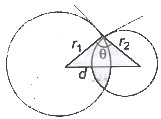Orthogonal Circles

Two circles are said to be intersect orthogonally, if their angle of intersection is a right angle.

If two circles

S1 ≡ x2 + y2 + 2g1x + 2f1y + C1 = 0 and

S2 ≡ x2 + y2 + 2g2x + 2f2y + C2 = 0 are orthogonal, then 2g1g2 + 2f1f2 = c1 + c2

Family of Circles

(i) The equation of a family of circles passing through the intersection of a circle x2 + y2 + 2gx + 2fy + c = 0 and line

L = lx + my + n = 0 is S + λL = 0

where, X, is any real number.

(ii) The equation of the family of circles passing through the point A(x1, y1) and B (x1, y1) is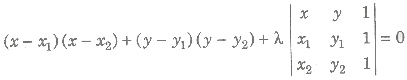(iii) The equation of the family of -circles touching the circle

S ≡ x2 + y2 + 2gx + 2fy + c = 0 at point P(x1, Y1) is

xx2 + y2 + 2gx + 2fy + c + λ, [xx1 + yy1 + g(x + x1) + f(Y+ Y1) + c] = 0 or S + λL = 0, where L = 0 is the equation of the tangent to

S = 0 at (x1, y1) and X ∈ R

(iv) Any circle passing through the point of intersection of two circles S1 and S2 is S1 +λ(S1— S2) = 0.

The radical axis of two circles is the locus of a point which moves in such a way that the length of the tangents drawn from it to the two circles are equal.

A system of circles in which every pair has the same radical axis is called a coaxial system of circles.

The radical axis of two circles S1 = 0 and S2 = 0 is given by S1 — S2 = 0.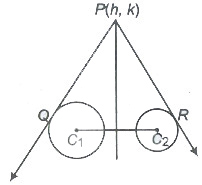1. The radical axis of two circles is always perpendicular to the line joining the centres of the circles.
2. The radical axis of three vertices, whose centres are non-collinear taken in pairs of concurrent.
3. The centre of the circle cutting two given circles orthogonally, lies on their radical axis.
4. Radical Centre The point of intersection of radical axis of three circles whose centre are non-collinear, taken in pairs, is called their radical centre.

Pole and Polar

If through a point P (x1, y1) (within or outside a circle) there be drawn any straight line to meet the given circle at Q and R, the locus of the point of intersection of tangents at Q and R is called the polar of P and po.:.at P is called the pole of polar.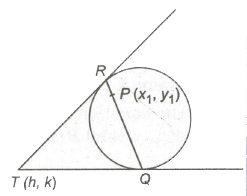1. Equation of polar to the circle x2 + y2 = r2 is xx1 + yy1 = r2.
2. Equation of polar to the circle x2 + y2 + 2gx + 2fy + c = 0 is xx1 + yy1 + g(x + x1) + f(y + y1) + c = 0
3. Conjugate Points Two points A and B are conjugate points with respect to a given circle, if each lies on the polar of the other with respect to the circle.
4. Conjugate Lines If two lines be such that the pole of one lies on the other, then they are called conjugate lines with respect to the given circle.

Coaxial System of Circles

A system of circle is said to be coaxial system of circles, if every pair of the circles in the system has same radical axis.

1. The equation of a system of coaxial circles, when the equation of the radical axis P ≡ lx + my + n = 0 and one of the circle of the system S = x2 + y2 + 2gx + 2fy + c = 0, is S + λP = 0.
2. Since, the lines joining the centres of two circles is perpendicular to their radical axis. Therefore, the centres of all circles of a coaxial system lie on a straight line, which is perpendicular to the common radical axis.

Limiting Points

Limiting points of a system of coaxial circles are the centres of the point circles belonging to the family.

Let equation of circle be x2 + y2 + 2gx + c = 0

∴ Radius of circle = √g2 — c

For limiting point, r = 0

∴ √g2 — c = 0 &rArr;g = ± √c

Thus, limiting points of the given coaxial system as (√c, 0) and (—√c, 0).

Important Points to be Remembered

(i) Circle touching a line L=O at a point (x1, y1) on it is

(x — x1)2 + (y — y1)2 + XL = 0.

(ii) Circumcircle of a A with vertices (x1, y1), (x2, y2), (x3, y3) is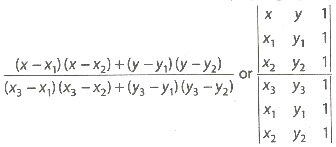(iii) A line intersect a given circle at two distinct real points, if the length of the perpendicular from the centre is less than the radius of the circle.

(iv) Length of the intercept cut off from the line y = mx + c by the circle x2 + y2 = a2 is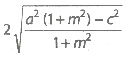(v) In general, two tangents can be drawn to a circle from a given point in its plane. If m1and m2 are slope of the tangents drawn from the point P(x1, y1) to the circle x2 + y2 = a2, then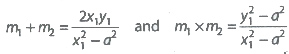(vi) Pole of lx + my + n = 0 with respect to x2 + y2 = a2 is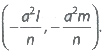(vii) Let S1 = 0, S2 = 0 be two circles with radii r1 , r2, then S1/r1 ± S2/r2 = 0 will meet at right angle.

(viii) The angle between the two tangents from (x1, y1) to the circle x2 + y2 = a2 is 2 tan-1 (a/√S1).

(ix) The pair of tangents from (0, 0) to the circle x2 + y2 + 2gx + 2fy + c = 0 are at right angle, if g2 + f2 = 2c.

(x) If (x1, y1) is one end of a diameter of the circle x2 + y2 + 2gx + 2fy + c = 0, then the other end will be (-2g – x1, -2f – y1).

Image of the Circle by the Line Minor

Let the circle be x2 + y2 + 2gx + 2fy + c = 0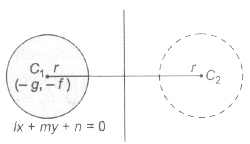and line minor lx + my + n = 0.

Then, the image of the circle is

(x — X1)2 + (y — y1)2 =r2

where, r = √g2 + f2 — c

Diameter of a Circle

The locus of the middle points of a system of parallel chords of a circle is called a diameter of the circle.

(i) The equation of the diameter bisecting parallel chords y = mx + c of the circle x2 + y2 = a2 is x + my = 0.

(ii) The diameter corresponding to a system of parallel chords of a circle always passes through the centre of the circle and is perpendicular to the parallel chords.

Common Tangents of Two Circles

Let the centres and radii of two circles are C1, C2 and r1, r2, respectively.

1. (i) When one circle contains another circle, no common tangent is possible.
Condition, C1C2 < r1 – r2
2. (ii) When two circles touch internally, one common tangent is possible.
Condition , C1C2 = r1 – r2
3. (iii) When two circles intersect, two common tangents are possible.
Condition, |r1 — r2| < C1C2 < |r1 + r2|
4. (iv) When two circles touch externally, three common tangents are possible.
Condition, C1C2 = r1 + r2
5. (v) When two circles are separately, four common tangents are possible.
Condition, C1C2 > r1 + r2

Important Points to be Remembered

Let AS is a chord of contact of tangents from C to the circle x2 + y2 = r2. M is the mid-point of AB.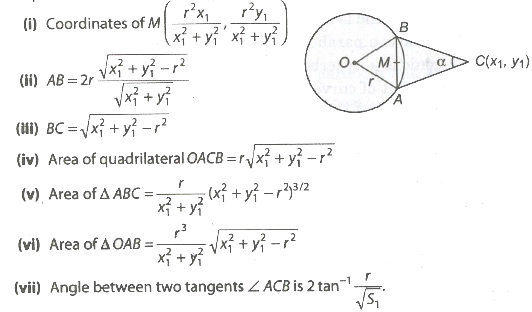Previous articleRectangular Axis & Straight Lines Notes Class 11th Maths
Next articleParabola Notes Class 11th Maths